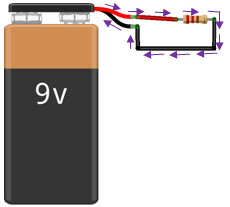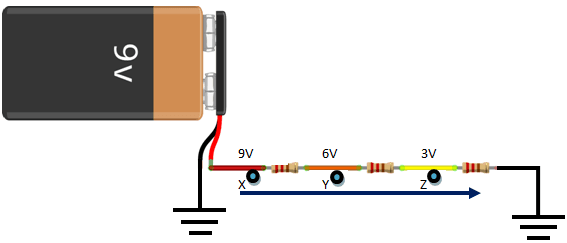# Current and Voltage – Relationship between current and voltage

Current and voltage are concepts in electronics that cannot be overemphasized in the process of electronic circuit design. However, to measure either of the two is one thing and to present the values in a comprehensive form is another thing.

In this tutorial, we are going to learn:

1. The concept of current
2. The concept of voltage
3. Current and voltage measurements
4. What power is in Electronics
5. Difference between Current and Voltage

Every electrical device you purchase has electrical parameter specifications, amongst them are current, voltage and power. It will sound awesome if I say I will skip the physics part of the concepts, but by doing so I would have missed out some important ideas that would help unravel the supposed mystery about the concept of current and voltage. Let’s explore these parameters intuitively, however, I will keep the basic physics involved in this tutorial to bare minimum.

## What is electric current? – current and voltage

Charges are carried by electrons. 1 electron charge is equal to -1.6 x10⁻¹⁹C.

-e = -1.6 x10⁻¹⁹C

Electrons which surround atoms carry charges as they move in their quantised states outside the nucleus of an atom. So, if an electron is caused to move in an atomic system, it carries with it “charge” entities of -1.6 x10⁻¹⁹C.

Let us say you have a system of charges carried by electrons confined within a boundary as shown in figure 1 below. If for any reason the charges are caused to move close to the boundary to enter side B from side A the number of charges that enter side B in one second gives one current unit. The symbol for current is I (capital letter) mostly used for direct current and i (small letter) used for alternating current.

Thus, if 10 charges cross and enter side B in 1 second, we have 10 current units. See figure 2 below:

Therefore, we have that Current = Charge/Time

Current = I
Charge = Q
Time = t

For example: I = Q/t = 10/1  = 10 current units.

If it took 2 seconds for the 10 charges to pass over to side B, it means that the number of charges that passed in 1 second will be 5.

Current = 10/2   = 5 current units.

The unit of current is called Ampere, Amp in short or just A. Hence: 10 current units = 10 Amperes or 10 Amps or 10 A.

## Conventional Current

Normally, we see current as flowing from the positive end of its supply source (e.g., battery) to the negative end.Figure 3: Current shown as moving from positive end to negative end of the battery.

From the diagram above, it looks like current is carried by positive charges moving from the positive end of the battery (shown in red color) to the negative end (shown in black color). The direction of current flow is indicated with purple arrows. But we know that current is created by charges in motion, and charges are carried by electrons which are negatively charged. The reason where we see current as being positive was as a result of past events..

Years back, before the electron was discovered; Benjamin Franklin termed any mysterious thing that moves and does work as being positively charged. But when J.J Thompson discovered that it was electrons that do the work in electronic circuits and they are not positively charged, the science community still continued with Benjamin Franklin’s convention, so, we call this virtual current “conventional current”.

But, bear in mind that electrons flow from the negative end to the positive end of the battery. Electrons are negatively charged and they are charge-carriers in batteries. It should be noted also that current is charge in motion. It’s only when a charge moves with time that we can refer to it as current. A charge that does not move can’t be called current.

## What is voltage? – current and voltage

These charges we talked about while explaining current do influence each other when they come close. If we have two charges that are both positively charged, the repel each other, i.e. they would not want to come much closer to each other. Same thing happens if they are both negatively charged. But if one is positively charged and the other negatively charged, they different charges will attract each other. In the different scenarios, either an attractive force or a repulsive force is experienced by the charges when they come close to each other.
Now, if you put a unit positive charge +Q in-between the negative charge denoted with –q and the positive charge denoted with +q, from the fact we established earlier, the unit positive charge +Q will be attracted to the –q and repelled by the +q. this unit positive charge will tend to move towards the negative charge -q and away from the positive charge +q.Figure 4: Influence of positive and negative charges on a unit positive charge.

When the above situation happens, the unit positive charge just moved from its initial position (point B) to another position (Point C), towards the direction of the negative charge –q. By moving a distance from Point B to Point C, it has done work. By doing work, it has used up energy with time. Hence, we conclude that the work a unit positive charge did in moving from Point B to Point C under the influence of surrounding charges (+q and –q) is called “Voltage” or electrical potential.

Voltage =  Electrical energy/Charge

Voltage = V
Energy = E
Charge = Q

The unit of Voltage is Volt, which is abbreviated as V.

So, if the charges in an electrical system did work by lighting up a bulb and by doing so exerted some electrical energy; if that energy is 10J and the number of charges that did the work is 2C, then, the voltage of the system will be:
V = E/Q = 10/2 = 5V.

## Potential Difference

When +Q was in-between –q and +q, it had greater force influence which made it to move to meet –q. when it met with –q (because unlike charges attract), that force pushing it from +q tends to zero. This means that it moved from a point of higher potential to a point of lower potential. Therefore, know from here that current which formed from charges can only move from regions of higher electrical potential or voltage to regions of lower electrical potential or voltage. This is like waterfall, water can only flow downwards.Figure 6: Current flow with respect to potential difference

From the figure above, point X is at 9V potential, point Y 6V potential and point Z 3V potential. The rule is this; current can only flow in the direction indicated by the dark blue arrow and not opposite it. This is because “current will only move from a region of higher voltage or electrical potential to a region of lower voltage or electrical potential. Voltage is the pressure moving current, and in fact, the Chinese refer to voltage as pressure.

## Current and Voltage Measurements

We can determine the value of current and voltage in an electronic circuit computationally or experimentally. By computation, we use mathematical equations or formulas; by experimentation we use measuring or test tools. Normally, the tool meant for measuring current is the ammeter, while the tool for measuring voltage is the voltmeter. However, a multitasking electronic parameter test tool called “Multimeter” can measure a bunch of electronic parameters with ease. All you need to do is set the multimeter to the parameter you want to measure.

I will make a tutorial on how to use a multimeter in the future, better still, you can buy the multimeter, when you buy it, you will get the self explanatory user manual that comes with it. The figure below is a labeled diagram of a digital multimeter DMM, you can turn the rotary switch’s pointer to any electronic parameter you want to measure which the multimeter has been designed to measure.

Since voltage is a potential, to measure any potential, we establish a reference point. In electronics, we take the natural ground or earth which has typically an infinite charge-absorbing ability to be a point with zero charge or current. Hence, it can serve a good reference point for comparison. In fact it is considered the 0-V (zero volts) reference point or ground point. The symbol for ground is shown below.

Nevertheless, sometimes in a circuit, voltages are specified without making reference to the ground. For example in the circuit below, we can say that potential difference or voltage from point A to point B is 4.5 volts, while the voltage from point A to point C is 9 volts. You can see that in the first case, we did not measure with reference to ground.

## What is Power in Electronics? – current and voltage

Generally, power is the time rate of doing work or the rate at which energy is dissipated with time. Power in electronics is the product of the interaction between current and voltage in a circuit, so, when we multiply the current in the circuit and the voltage that drives it, we obtain the power.

Power = V×I

We know that V=E/Q , I =Q/T

Power = V×I = E/Q × Q/T = E/T = Energy dissipated with time or time rate of energy dissipated to do work. The formula can also be
P = Work/Time = W/T

There is more to learn about power which we shall discuss after we have treated Resistance and resistors.

## Summary:

• Current is the time rate of flow of charges in a circuit
• Voltage is the electronic potential difference between two points in a circuit.
• Current moves from region of higher electronic potential to a region of lower electronic potential
• Multimeter is an electronic tool used to measure various electronic parameters
• The natural ground which has infinite current absorbing ability is the best reference to compare and measure voltages
• Power is the product of voltage and current in a circuit.

Assignment:

Get a digital and an analog multimeter form amazon , use them to measure same voltage points, what difference could you observe in using the two different multimeters? Which one do you prefer most and for what reasons?